﻿ Does Anyone Need a GARCH(1,1)?

Does Anyone Need a GARCH(1,1)?

Erhard ReschenhoferOPEN ACCESSPEER-REVIEWED

Does Anyone Need a GARCH(1,1)?

Erhard ReschenhoferDepartment of Statistics and Operations Research, University of Vienna, Vienna, Austria

Abstract

Hansen and Lunde  posed the question Does anything beat a GARCH(1,1)? and compared a large number of parametric volatility models in an extensive empirical study. They found that no other model provides significantly better forecasts than the GARCH(1,1) model. In contrast, this paper arrives at the conclusion that simple robust estimators such as weighted medians of past (squared) returns outperform the GARCH(1,1) model both in-sample as well as out-of-sample. This conclusion is based on theoretical arguments as well as on empirical evidence.

At a glance: Figures

123
Prev Next

• Reschenhofer, Erhard. "Does Anyone Need a GARCH(1,1)?." Journal of Finance and Accounting 1.2 (2013): 48-53.
• Reschenhofer, E. (2013). Does Anyone Need a GARCH(1,1)?. Journal of Finance and Accounting, 1(2), 48-53.
• Reschenhofer, Erhard. "Does Anyone Need a GARCH(1,1)?." Journal of Finance and Accounting 1, no. 2 (2013): 48-53.

 Import into BibTeX Import into EndNote Import into RefMan Import into RefWorks

1. Introduction

The estimation and forecasting of the volatility of asset returns is a key issue for risk management, portfolio allocation, and option pricing. Poon and Granger [Journal of Economic Literature, 51, 478-539, 2003.">27, Financial Analysts Journal, 61, 45-56, 2005.">28], Poon , Andersen et al. , and Knight and Satchel  provide extensive reviews of the main theoretical and empirical findings. The parametric volatility models proposed in the literature include autoregressive conditional heteroscedasticity (ARCH) , generalized ARCH (GARCH) , integrated GARCH (IGARCH) , logarithmic GARCH (log-GARCH) [Econometric Review, 5, 57-61, 1986.">14, Econometric Review, 5, 71-74, 1986.">24], absolute value GARCH (AVGARCH) [Journal of Finance, 44, 1115-1153, 1989.">31, 33], exponential GARCH (EGARCH) , non-linear GARCH (NGARCH) , asymmetric power ARCH (APARCH) , threshold GARCH (TGARCH) , generalized quadratic GARCH (GQARCH)  and augmented GARCH (Aug-GARCH)  models as well as the models suggested by Engle and Ng , Glosten et al. , and Hentshel . Hansen and Lunde  found that none of these models provides a significantly better forecast than the simple GARCH(1,1) model. In their comparison, they used sums of squared intraday returns instead of squared daily returns for the evaluation of the forecasting performance because the latter quantities are only poor proxies for daily volatilities. The use of high-frequency data was proposed by Anderson and Bollerslev  in order to resolve the apparent contradiction between the poor predictive power of parametric volatility models for squared daily returns and the highly significant in-sample parameter estimates [Journal of Derivatives, Winter, 51-63, 1993.">5, Financial Markets, Institutions and Instruments 6, 1-88, 1997.">12, Journal of Finance, 50, 507-528, 1995.">20, The Microstructure of Foreign Exchange Markets, Frankel, J.A., Galli, G. and Giovannini, A. (eds), The University of Chicago Press, 19-37, 1996.">21].

The usefulness of the GARCH(1,1) model is challenged in the next two sections. Section 2 comments on some fundamental disadvantages of this simple model and proposes possible further developments. Section 3 points out that the existing empirical evidence of its poor forecasting performance cannot be explained entirely by the use of a noisy volatility proxy. Finally, Section 4 provides empirical evidence of its poor in-sample performance. It is shown that simple robust estimators such as weighted medians of past (squared) returns clearly outperform the GARCH(1,1) model. Section 5 concludes.

2. Modeling Volatility

If daily stock returns xt are stationary, their unconditional variance σ2=E(xt-μ)2 can be estimated simply by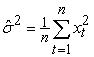(1)

because the mean return μ is negligible relative to σ2. However, plotting squared or absolute returns against time reveals prominent volatility fluctuations. For illustration, Figure 1.a shows such plots for nine components of the Dow Jones Industrial Average. The sole criterion for the selection of the nine stocks was the availability of a long series of historical prices at Yahoo!Finance. The selected stocks are Alcoa (AA), Boeing (BA), Caterpillar (CAT), Du Pont (DD), Walt Disney (DIS), General Electric (GE), Hewlett-Packard (HPQ), IBM (IBM), and Coca-Cola (KO). Their prices are available since January 2, 1962. The sample period ends on April 12, 2013. The observed volatility fluctuations may be due to deviations from stationarity, e.g., structural breaks in the unconditional variance. Another possible explanation is that squared returns are serially correlated. In the latter case, the conditional variances could be modeled in terms of past observations, e.g.,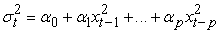(2)

and the returns by(3)

where the random variables zt are independent and identically distributed with mean zero and unit variance (ARCH(p) model ). Often a more parsimonious model can be obtained by using(4)

instead of (2) (GARCH(p,q) model ).

The naive use of the statistics(5)

corroborates that squared returns are indeed autocorrelated (see Figure 2.a). However, the fact that these statistics involve fourth powers of returns poses a problem. Some returns are always much larger than the others. If returns are raised to the fourth power, the smaller returns will be practically zero compared to the larger returns. Even in case of large n, ρk will therefore depend critically on a very small number of large returns. An easy way to overcome this problem is to use absolute returns rather than squared returns. However, there is another serious problem which cannot be solved by switching to absolute returns. A comparison of Figures 1.a and 1.b shows that clusters of large and small squared returns, respectively, are more noticeable in longer time periods than in shorter time periods. In shorter time periods, it is not even clear whether there is any autocorrelation at all (see Figure 2.b). Figure 3 is similar to 2. The only difference is that absolute returns were used instead of squared returns. The patterns shown in Figure 3 are therefore more reliable. However, there is still hardly any evidence of autocorrelation in shorter time periods.

Figure 1. Absolute daily returns of nine components of the Dow Jones Industrial Average: AA (red), BA (pink), CAT (orange), DD (gold), DIS (brown), GE (green), HPQ (blue), IBM (gray), KO (black)

There are two possibilities. Either there is no short-term autocorrelation or the size of the return is a bad proxy for volatility. It is easy to see that the second possibility is the more likely. A severe disadvantage of the daily return is that even after a trading day with extreme fluctuations the closing price might by chance be close to the previous closing price, which would be interpreted as an indication of low volatility. An obvious alternative is to use the intraday range as a proxy for volatility. Figure 4 shows that in this case there is no doubt about the presence of conditional heteroskedasticity. But since the autocorrelation function appears to decay much slower than in the case of a GARCH(1,1) model (see Figure 2.a), it is questionable whether this model is also suitable for the description of the long-term fluctuations in volatility. Long-memory volatility models or volatility break models are probably better suited for the latter task (for a discussion of these models see , pp. 45-58).

We conclude that conditional heteroskedasticity models are, in principle, appropriate but the specifications (2) and (4) are inappropriate. Possible alternatives are given by(6)

and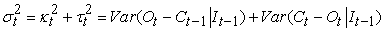(7)

where It-1 is the sigma field generated by the past information until time t-1, Jt=OtCt-1 is the opening jump, Rt=HtLt is the intraday range, and Ot, Ht, Lt, and Ct are the (log) opening, high, low, and closing prices at time t. The investigation of univariate specifications such as (6) and bivariate specifications such as (7) is beyond the scope of this paper and must be left for future research. A simple special case of (6) is obtained by choosing p=2 and α2=0. While this choice may be convenient for theoretical investigations, the choice p=1 and α1=0 would probably be more useful from an applied point of view. The simplest bivariate approach would be to apply an MGARCH model to the bivariate process (Jt,Rt)T (for a survey of multivariate GARCH models see ). However, it remains to be seen how well this simple approach will work in practice.

3. Volatility Measures

The fact that the returns xttzt contain only very limited information about σt (because of the variability of zt) does, of course, not imply that they are of no use for volatility forecasting. The weighting is crucial. GARCH models, which assign very high weights to the most recent (squared) returns, perform much worse than simple robust statistics such as weighted medians of past returns . Note that the median of the squared returns is identical to the squared median of the absolute returns. It is therefore irrelevant whether absolute values or squares are used. The bad performance of GARCH models is not surprising in the light of the observation that short-term correlation between squared returns is very weak (Figure 2.b). It would be naive to expect that their competitive position will improve dramatically if more reliable proxies for the conditional variance are used instead of the squared return for the evaluation of the forecasting performance. After all, the squared return is an (approximately) unbiased estimator for the conditional variance and the sample sizes in empirical studies of daily financial data are usually extremely large. In this context, supporters of GARCH models often refer to Hansen and Lunde  who showed that the substitution of a squared return for the conditional variance in the evaluation of volatility models can result in inconsistent rankings. However, these authors warned in particular of using the criterion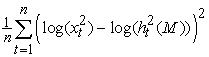(8)

for evaluating the forecasts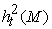obtained from model M, while many empirical rankings (e.g., ) are based on criteria such as(9)

which are free from suspicion. Furthermore, more sophisticated proxies which are based on intraday statistics are typically severely biased. The only exception is the trivial estimator(10)

If the second term is replaced by the squared intraday range ([The Journal of Business, 53, 67-78, 1980.">13, The Journal of Business, 53, 61-65, 1980.">25]) or the sum of squared intraday returns (realized volatility; see [International Economic Review, 39, 885-905, 1998.">1, Journal of Applied Econometrics, 20, 873-889, 2005.">16]), each term must be multiplied by unknown weights to achieve unbiasedness. A major problem with this approach is that these weights are not constant. They differ between different stocks and also between different time periods .

Up to now, forecasts of the conditional variance and the conditional standard deviation, respectively, have been discussed which are obtained from information up to time t-1. In the following, the focus will be on estimates of the conditional standard deviation which are obtained from information up to time t. These estimates will be used for the normalization of the intraday range. To avoid any problems with the unknown weights mentioned above, the differences CtOt will be examined instead of the daily returns CtCt-1. If an estimate of the conditional standard deviation of CtOt is accurate, the distribution of the intraday range divided by this estimate can be approximated by that of the range of a standard Brownian motion. Figure 5 compares a histogram of intraday ranges divided by the global sample standard deviation of the differences CtOt with the asymptotic density function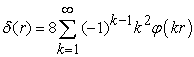(11)

of the range of a standard Brownian motion on [0,1] , where denotes the standard normal density function. Not surprisingly, the agreement is poor because volatility fluctuations have not been taken into account. A slight improvement is obtained when the global sample standard deviation is replaced by a local one which is based on the last m=200 values (see Figure 6).

Using the more robust estimate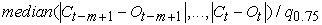(12)

where q0.75 is the 0.75 quantile of the standard normal distribution, results in a further improvement (see Figure 7). The best results are obtained when weighted medians (with exponentially decaying weights ∝ 0.99j, j=0,...,m-1) are used (see Figure 8). Again, the GARCH(1,1) estimates (obtained from samples of size m) cannot keep up (see Figure 9).

Figure 5. Histogram of intraday range (divided by global standard deviation) versus asymptotic density function of the range of a standard Brownian motion (sample period: 1962.01.02 - 2013.04.12)
Figure 6. Histogram of intraday range (divided by local standard deviation) versus asymptotic density function of the range of a standard Brownian motion (sample period: 1962.01.02 - 2013.04.12)
Figure 7. Histogram of intraday range (rescaled with local median) versus asymptotic density function of the range of a standard Brownian motion (sample period: 1962.01.02 - 2013.04.12)
Figure 8. Histogram of intraday range (rescaled with weighted median) versus asymptotic density function of the range of a standard Brownian motion (sample period: 1962.01.02 - 2013.04.12)

5. Conclusion

The empirical evidence presented in this paper challenges the use of squared or absolute returns for the modeling of daily return volatility. If intraday statistics are used instead, short-term conditional heteroskedasticity becomes much more visible. Accordingly, univariate and bivariate models which are based on common intraday statistics are proposed as alternatives to GARCH models which are based on squared returns only.

Studies questioning the usefulness of GARCH models are often discredited by referring to Hansen and Lunde  who have warned that the use of the squared return as a proxy for conditional variance can result in inconsistent rankings of volatility models. It is pointed out that this warning does not apply to a great deal of the sceptical studies and also that alternative proxies have their own disadvantages.

Finally, empirical evidence is provided which shows that not only the out-of-sample performance of the GARCH(1,1) model is bad but also its in-sample performance. These findings corroborate the results of previous studies focussing on volatility forecasting (e.g., ) which suggest that the GARCH(1,1) model cannot keep up with simple robust estimators such as weighted medians. The evidence of its poor in-sample performance presented in Section 4 is quite strong because its interpretation depends only on the assumption that the distribution of the intraday range can be approximated by that of the range of a Brownian motion.

Figure 9. Histogram of intraday range (rescaled with GARCH(1,1) model) versus asymptotic density function of the range of a standard Brownian motion (sample period: 1962.01.02 - 2013.04.12)

References

  Andersen, T.G. and Bollerslev, T., "Answering the skeptics: yes, standard volatility models do provide accurate forecasts," International Economic Review, 39, 885-905, 1998.In article CrossRef  Andersen, T. G., Bollerslev, T., Christoffersen, P. F., and Diebold, F. X., "Volatility and correlation forecasting," in Handbook of Economic Forecasting, Elliott, G., Granger, C.W.J. and Timmermann, A. (eds), North-Holland, Amsterdam, 2006.In article  Bauwens, L., Laurent, S. and Rombouts, J.V.K., "Multivariate GARCH models: A survey," Journal of Applied Econometrics, 21, 79-109, 2006.In article CrossRef  Bollerslev, T., "Generalized autoregressive conditional hetero-skedasticity," Journal of Econometrics, 31, 307-327, 1986.In article CrossRef  Cumby, R., Figlewski S. and Hasbrouck, J. "Forecasting Volatility and Correlations with EGARCH Models," Journal of Derivatives, Winter, 51-63, 1993.In article CrossRef  Ding, Z., Granger, C.W. J. and Engle, R.F., "A long memory property of stock market returns and a new model," Journal of Empirical Finance, 1, 83-106, 1993.In article CrossRef  Duan, J., "Augmented GARCH(p, q) process and its diffusion limit," Journal of Econometrics, 79, 97-127, 1997.In article CrossRef  Engle, R.F., "Autoregressive conditional heteroscedasticity with estimates of the variance of United Kingdom inflation," Econometrica, 50, 987-1007, 1982.In article CrossRef  Engle, R.F. and Bollerslev, T., "Modelling the persistence of conditional variances,” Econometric Reviews, 5, 1-50, 1986.In article CrossRef  Engle, R.F. and Ng, V., "Measuring and testing the impact of news on volatility," Journal of Finance, 48, 1747-1778, 1993.In article CrossRef  Feller, W., "The asymptotic distribution of the range of sums of independent random variables," The Annals of Mathematical Statistics, 22, 427-432, 1951.In article CrossRef  Figlewski, S., "Forecasting Volatility," Financial Markets, Institutions and Instruments 6, 1-88, 1997.In article CrossRef  Garman, M.B. and Klass, M.J., "On the estimation of security price volatilities from historical data", The Journal of Business, 53, 67-78, 1980.In article CrossRef  Geweke, J., "Modelling persistence in conditional variances: A comment," Econometric Review, 5, 57-61, 1986.In article CrossRef  Glosten, L. R., Jagannathan, R. and Runkle, D.E., "On the relation between the expected value and the volatility of the nominal excess return on stocks," Journal of Finance, 48, 1779-1801, 1993.In article CrossRef  Hansen, P.R. and Lunde, A., "A forecast comparison of volatility models: does anything beat a GARCH(1,1)?," Journal of Applied Econometrics, 20, 873-889, 2005.In article CrossRef  Hansen, P.R. and Lunde, A., "Consistent ranking of volatility models," Journal of Econometrics, 131, 97-121, 2006.In article CrossRef  Hentshel, L., "All in the family: Nesting symmetric and asymmetric GARCH models," Journal of Financial Economics, 39, 71-104, 1995In article CrossRef  Higgins, M.L. and Bera, A.K., "A class of nonlinear ARCH models," International Economic Review, 33, 137-158, 1992.In article CrossRef  Jorion, P., "Predicting Volatility in the Foreign Exchange Market," Journal of Finance, 50, 507-528, 1995.In article CrossRef  Jorion, P., "Risk and Turnover in the Foreign Exchange Market," in The Microstructure of Foreign Exchange Markets, Frankel, J.A., Galli, G. and Giovannini, A. (eds), The University of Chicago Press, 19-37, 1996.In article  Knight, J. and Satchell, S. (eds), Forecasting Volatility in the Financial Markets, Butterworth-Heinemann, 3rd edition, 2007.In article  Nelson, D.B., "Conditional heteroskedasticity in asset returns: A new approach," Econometrica, 59, 347-370, 1991.In article CrossRef  Pantula, S.G., "Modelling persistence in conditional variances: A comment," Econometric Review, 5, 71-74, 1986.In article CrossRef  Parkinson, M., "The extreme value method for estimating the variance of the rate of return," The Journal of Business, 53, 61-65, 1980.In article CrossRef  Poon, H., A practical guide to forecasting financial market volatility, Wiley, 2005.In article  S. Poon, C.W.J. Granger, "Forecasting volatility in financial markets: a review," Journal of Economic Literature, 51, 478-539, 2003.In article CrossRef  S. Poon, C.W.J. Granger, 2005. "Practical issues in forecasting volatility," Financial Analysts Journal, 61, 45-56, 2005.In article CrossRef  Reschenhofer, E., "Forecasting volatility: double averaging and weighted medians," International Journal of Computational Economics and Econometrics, 1. 317-326. 2010.In article CrossRef  Reschenhofer, E., "Using intraday statistics for the estimation of the return variance," Journal of Finance and Investment Analysis, 2, 1-13, 2013.In article  Schwert, G.W., "Why does Stock volatility change over time?," Journal of Finance, 44, 1115-1153, 1989.In article CrossRef  Sentana, E., "Quadratic ARCH models," Review of Economic Studies, 62, 639-661, 1995.In article CrossRef  Taylor, S.J., Modelling Financial Time Series. John Wiley & Sons, 1986.In article  Zakoian, J.-M., "Threshold heteroskedastic models," Journal of Economic Dynamics and Control, 18, 931-955, 1994.In article